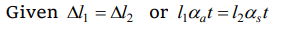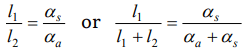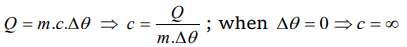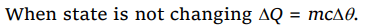## Thermal Properties of Matter Questions and Answers Part-7

1. Two rods, one of aluminum and the other made of steel, having initial length $l_{1}$   and $l_{2}$  are connected together to form a single rod of length $l_{1} + l_{2}$   . The coefficients of linear expansion for aluminum and steel are $\alpha_{a}$  and $\alpha_{s}$  respectively. If the length of each rod increases by the same amount when their temperature are raised by to C , then find the ratio $\frac{l_{1}}{l_{1}+l_{2}}$
a) $\frac{\alpha_{s}}{\alpha_{a}}$
b) $\frac{\alpha_{a}}{\alpha_{s}}$
c) $\frac{\alpha_{s}}{\left(\alpha_{a}+\alpha_{s}\right)}$
d) $\frac{\alpha_{a}}{\left(\alpha_{a}+\alpha_{s}\right)}$

Explanation:2. When vapour condenses into liquid
a) It absorbs heat
b) It liberates heat
c) Its temperature increases
d) Its temperature decreases

Explanation: In vapor to liquid phase transition, heat liberates.

3. At NTP water boils at 100°C. Deep down the mine, water will boil at a temperature
a) 100°C
b) > 100°C
c) < 100°C
d) Will not boil at all

Explanation: Pressure inside the mines is greater than that of normal. Pressure. Also we know that boiling point increases with increase in pressure.

4. If specific heat of a substance is infinite, it means
a) Heat is given out
b) Heat is taken in
c) No change in temperature takes place whether heat is taken in or given out
d) All of the above

Explanation:5. A gas in an airtight container is heated from 25°C to 90°C. The density of the gas will
a) Increase slightly
b) Increase considerably
c) Remain the same
d) Decrease slightly

Explanation: Mass and volume of the gas will remain same, so density will also remain same

6.A quantity of heat required to change the unit mass of a solid substance, from solid state to liquid state, while the temperature remains constant, is known as
a) Latent heat
b) Sublimation
c) Hoar frost
d) Latent heat of fusion

Explanation: Latent heat of fusion

7. The latent heat of vaporization of a substance is always
a) Greater than its latent heat of fusion
b) Greater than its latent heat of sublimation
c) Equal to its latent heat of sublimation
d) Less than its latent heat of fusion

Explanation: The latent heat of vaporization is always greater than latent heat of fusion because in liquid to vapour phase change there is a large increase in volume. Hence more heat is required as compared to solid to liquid phase change

8.The factor not needed to calculate heat lost or gained when there is no change of state is
a) Weight
b) Specific heat
c) Relative density
d) Temperature change

Explanation:9. 540 g of ice at 0°C is mixed with 540 g of water at 80°C. The final temperature of the mixture is
a) 0°C
b) 40°C
c) 80°C
d) Less than 0°C